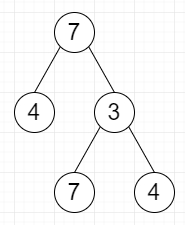# Counting Maximal Value Roots in Binary Tree in C++

C++Server Side ProgrammingProgramming

Suppose we have a binary tree root; we have to count the number of nodes where its value is greater than or equal to the values of all of its descendants.

So, if the input is likethen the output will be 4 as all nodes except 3, it meets the criteria.

To solve this, we will follow these steps −

• Define a function dfs(), this will take node,

• if node is not null, then −

• return 0

• l := dfs(left of node)

• r := dfs(right of node)

• if val of node >= maximum of l and r, then −

• (increase ret by 1)

• x := maximum of val of node, l and r

• return x

• From the main method, do the following,

• ret := 0

• dfs(root)

• return ret

Let us see the following implementation to get better understanding −

## Example

Live Demo

#include <bits/stdc++.h>
using namespace std;
class TreeNode {
public:
int val;
TreeNode *left, *right;
TreeNode(int data) {
val = data;
left = NULL;
right = NULL;
}
};
class Solution {
public:
int ret;
int dfs(TreeNode* node){
if(!node)
return 0;
int l = dfs(node->left);
int r = dfs(node->right);
if(node->val >= max(l, r)) {
ret++;
}
int x = max({node->val, l, r});
return x;
}
int solve(TreeNode* root) {
ret = 0;
dfs(root);
return ret;
}
};
main(){
Solution ob;
TreeNode *root = new TreeNode(7);
root->left = new TreeNode(4);
root->right = new TreeNode(3);
root->right->left = new TreeNode(7);
root->right->right = new TreeNode(5);
cout << (ob.solve(root));
}

## Input

TreeNode *root = new TreeNode(7);
root->left = new TreeNode(4);
root->right = new TreeNode(3);
root->right->left = new TreeNode(7);
root->right->right = new TreeNode(5);

## Output

4